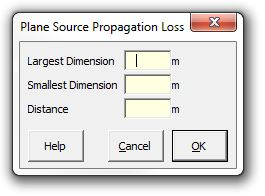### Strutt Help

Plane Source Propagation Loss    1/1,1/3

Strutt|Propagation|Plane Source calculates the Sound Pressure Level resulting from the Sound Power Level of a plane source (e.g. a duct opening or a building wall), given the dimensions of the source (m) and the distance from the source (m). The source is assumed to be radiating into hemispherical space.The radiation of noise from an plane source of noise encompasses three main regions: the near field close to the source, an intermediate zone where the source acts as a line source, and the distant zone where the source behaves as a point source. Due to the (assumed) location of the plane source on a reflective surface in the far-field the plane source approaches hemispherical radiation.

The distance correction from the plane source is calculated using Equation 5.105 of Bies and Hansen (3rd Edition):

(:p^2:)=(2pcW)/(piHL) tan^(-1)((HL)/(2r sqrt(H^2+L^2+4r^2)))
L_p = L_W-10log_10(HL)+10log_10[tan^(-1)((HL)/(2r sqrt(H^2+L^2+4r^2)))]-2

In the near field (approximately r < a//pi), the sound level can be approximated as:

L_p=L_W-10log_10 S+DI

In the line-source intermediate region (approximately 2a//pi < r < 2b//pi) , the sound level can be approximated as:

L_p=L_W-10log_10 S-10log_10(r/(a//pi))+DI

In the point-source far region (approximately r > 2b//pi), the sound level can be approximated as:

L_p=L_W-10log_10 S+10log_10(a/b)-20log(r/(b//pi))+DI

where r is the distance from the source, H and L are the minor and major source dimensions (m) (the smaller dimension is further defined as a and the larger dimension as b), S=H*L is the area of the source (m˛) and DI is the directivity index of the source (dB).